# Dynamic tsunami generation due to sea-bottom deformation: Analytical representation based on linear potential theory

## Abstract

Recording ocean-bottom pressures in offshore regions has increased our understanding of tsunami sources and promoted the development of rapid source estimation for early tsunami warning. Analytical solutions for the water height at the surface have often played an important role as a reference model in these efforts. To understand the system better and to develop the techniques further, not only the water height but the velocity in the sea and the pressure at the sea bottom are important. The present study obtains a solution for the velocity potential for sea-bottom deformation with an arbitrary source time function, and derives analytical solutions for the velocity distributions in the sea and the pressure at the bottom. These enable us to visualize the tsunami generation process, including the velocity field. The solutions can also give a theoretical background for setting the initial conditions of height and velocity in 2-D tsunami propagation simulations. Furthermore, the solution of the ocean-bottom pressure indicates that when the sea-bottom uplifts at an increasing rate, the sea-bottom pressure increases through a dynamic effect. This dynamic contribution will not be negligible as we develop more rapid and precise source estimation techniques using ocean-bottom pressure gauges within the source region.

## 1. Introduction

The tsunami generation/propagation problem has been a core focus of tsunami research. Laboratory experiments can accurately control the size and duration of the tsunami source and can observe the generation and propagation processes in situ, which is very useful for studying the fundamental fluid dynamics of tsunamis (e.g., Hammack, 1973). Since a limited number of observation stations exist in the vast ocean, and tsunamis are usually recorded at stations far from the source region, waveforms observed inside the source region are rarely analyzed, and the nature of tsunamis are difficult to determine in detail. On 11 March, 2011, a huge MW 9.1 earthquake, known as the 2011 Tohoku-Oki earthquake, occurred off the Pacific coast of northeastern Honshu, Japan, where numerous sensors, such as seismometers, GPS stations, thermometers, and pressure gauges, were deployed (e.g., Ito et al., 2011; Sato et al., 2011). By analyzing the tsunami waveforms, in particular, those recorded inside the source region, Saito et al. (2011) estimated the tsunami source with high resolution. Also, after the 2011 Tohoku-Oki earthquake, the National Research Institute for Earth Science and Disaster Prevention (NIED) started to construct a dense ocean-bottom network in and around the source region of the Tohoku earthquake (Fig. 1) in order to detect seismic and tsunami signals more rapidly, and to contribute to more reliable seismic and tsunami warnings in the case of future gigantic earthquakes (e.g. Kanazawa and Shinohara, 2009; Monastersky, 2012; Uehira et al., 2012; Kanazawa, 2013). In order to fully interpret nearfield tsunami records or the sea-bottom pressure within the source region, theoretical models that can correctly include the tsunami generation process are very important.

Numerical simulation using a high-performance computer offers a method for theoretically calculating tsunami generation and propagation (e.g., Mader, 2004). Two-dimensional numerical simulation, using linear long-wave, linear dispersive, or non-linear tsunami equations, is a good choice for proper tsunami-propagation modeling (e.g., Shuto, 1991; Liu, 2009; Cui et al., 2010; Saito et al., 2010). Three-dimensional simulation is suitable when investigating tsunami generation due to sea-bottom deformation, because it is necessary to correctly simulate the vertical flow over the sea depth (Ohmachi et al., 2001; Grilli et al., 2002; Kakinuma and Akiyama, 2006). Saito and Furumura (2009) investigated tsunami generation due to sea-bottom deformation by numerically solving the 3-D equations of motion. Nosov and Kolesov (2007) considered the compressibility of the fluid and synthesized the sea-bottom pressure records for the 2003 Tokachi-Oki earthquake. Maeda and Furumura (2013) developed a code in a framework of 3D elastic equations of motion, which naturally simulates both seismic and tsunami waves, including their coupling effects. Dutykh et al. (2013) investigated the spatial and temporal distribution of water height during tsunami generation based on linear and nonlinear theories.

In order to confirm the validity of the numerical simulations, and to interpret complicated simulated or observed waveforms, analytical solutions provide a good reference. The linear theory has been developed as a fundamental theory related to the generation/propagation problem (e.g., Lamb, 1932; Takahashi, 1942; Stoker, 1958; Leblond and Mysak, 1978; Mei, 1989; Ward, 2001). Using linear potential theory, in which the velocity potential is introduced and wave linearity is assumed, the spatial and temporal change of the surface height due to bottom deformation are well reproduced in many instances, as observed in laboratory experiments and calculated in numerical simulations (e.g., Hammack, 1973; Kervella et al., 2007). One of the notable consequences of the theory is that the height distribution at the surface is not always identical to the bottom deformation (e.g., Kajiura, 1963; Ward, 2001). Some studies take this difference into account in order to calculate an initial tsunami-height distribution for 2-D tsunami propagation simulations (e.g., Tanioka and Seno, 2001). Saito and Furumura (2009) interpreted the relationship between sea-bottom deformation and the initial tsunami-height distribution as two spatial lowpass-filter processes, in which the cut-off wavenumbers are given by the sea depth and the source duration. By taking these effects into account, and using the analytical solution for the water height, Tsushima et al. (2012) carefully investigated the ocean-bottom pressure records within the source region. In many past studies, the excess pressure at the sea bottom due to a tsunami is usually assumed to be given by a static contribution from the tsunami height; for instance, given by ρ0g0η, where ρ0 is the density of sea water, g0 is the gravitational constant and η is the tsunami height. However, this approximation is not always valid. It is important to show the quantitative relationship, or the difference between tsunami height and the pressure at the bottom for more rigorous analyses.

Theoretical studies on tsunami generation have focused mainly on the water height at the surface (e.g. Kajiura, 1963; Dutykh, 2013). Few studies have investigated the veocity or displacement field in the sea during the tsunami generation. An exception was Ward (unpublished docment available at http://es.ucsc.edu/̃ward/papers/Basic_ Tsunami Theory.doc), in which the solution of the displacement in the sea was theoretically derived for instantaneous sea-bottom uplift. Previous studies have the limitation that the mathematical expression of the velocity potential in the time domain has not yet been obtained for a sea-bottom deformation characterized by an arbitrary source time function. The solution has only been obtained through a formal expression using an inverse Fourier/Laplace transform, or for very limited situations. Dutykh and Dias (2007) and Kervella et al. (2007) theoretically derived the velocity distribution only at the sea surface, but not over the sea depth. They also derived the pressure at the sea bottom, but only after an instantaneous sea-bottom deformation. Levin and Nosov (2009) indicate the velocity potential in the time domain for a very special case of the 1-D sea-bottom deformation characterized by constant-velocity uplift.

The velocity potential distribution in the sea is critically important in order to fully understand the generation process. Also, the sea-bottom pressure during tsunami generation is critically important for developing a tsunami early warning because the sea-bottom pressure, rather than the water height, is currently measurable inside the source region (e.g., Tsushima et al., 2012). However, analytical solutions for the velocity in the sea and the pressure at the sea bottom have not yet been completed. This limitation comes from the fact that the velocity potential in the time domain has not generally been obtained.

The purpose of the present study is to derive a mathematical expression for the velocity potential in the time domain for a sea-bottom deformation characterized by an arbitrary source time function based on the linear potential theory. Using this mathematical expression, we can investigate the tsunami generation process from the viewpoint of velocity distribution and ocean-bottom pressure, in addition to the water height at the surface. In Section 2, we formulate a linear potential theory and obtain mathematical expressions for the velocity potential. We also derive analytical solutions for the pressure at the bottom and the velocity distribution in the sea. We then investigate the sea-bottom pressure during tsunami generation. In Section 3, we interpret the solutions we derived in the previous section in terms of the tsunami generation and propagation processes. We also theoretically investigate the initial conditions for 2-D tsunami simulations based on the equations we have derived. In Section 4, as an example, we plot the water height at the surface and the velocity distributions from the sea-bottom deformation on large and small sizes. Finally, in Section 5, we present concluding remarks.

## 2. Linear Potential Theory

### 2.1 A general framework

We formulate tsunami generation and propagation from sea-bottom deformation in a constant water depth based on linear potential theory (e.g., Takahashi, 1942; Hammack, 1973). We use the Cartesian coordinates shown in Fig. 2, where the z-axis is vertically upward, and the x- and y-axes lie in a horizontal plane. The sea surface is located at z = 0, and the sea bottom is flat and located at z = −h0. We assume that the height of the sea surface η (x, y, t) at time t is small enough compared with the water depth ǀηǀ h0, and viscosity is neglected. The velocity in the fluid is given by a vector v (x, t) = v x e x + v y e y + v z e z , where x = xe x + yey + ze z , and e x , e y , and e z are the basis vectors in the x-, y-, and z-axes, respectively. We also assume an incompressible and irrotational flow, v = 0, in which the velocity vector is given as v (x, t) = gradφ (x, t) using a velocity potential φ (x, t).

The velocity potential satisfies the Laplace equation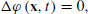((1))

and the boundary conditions at the surface (z = 0) are given by((2))((3))

where g0 is the gravitational constant. Equation (2) is a linearized dynamic boundary condition indicating that the pressure is constant along the sea surface, and Eq. (3) is a linearized kinematic boundary condition indicating that the sea surface does not break during the motion (e.g. Lamb, 1932; Stoker, 1958). Assuming the final sea-bottom deformation, or the permanent vertical displacement at the sea bottom, to be d(x, y), we give the vertical component of the velocity as the boundary condition at the sea bottom, as follows:((4))

where the function χ (t) depends only on time, and satisfies the following: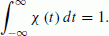((5))

The function χ (t) has the dimension of the inverse of time, and is referred to hereinafter as the source time function. We obtained the velocity potential that satisfies (1), (2), (3), and (4) as follows (e.g., Saito and Furmura, 2009):((6))

where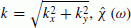is the Fourier transform in the time-frequency domain defined as follows:((7))

andis the 2-D Fourier transform in the space-wavenumber domain defined as follows: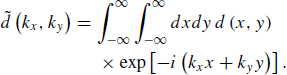((8))

Equation (6) represents a formal expression of the velocity potential with the inverse Fourier transform with respect to the time-frequency domain. This was derived by Takahashi (1942) in cylindrical coordinates. Similar equations were obtained by Kervella et al. (2007) and Levin and Nosov (2009) for the Cartesian coordinates using the inverse Laplace transform. It is necessary to integrate over the angular frequency ω in order to obtain a solution in the time domain. Takahashi (1942) and Kervell et al. (2007) calculated the integral for the sea surface z = 0, but not for an arbitrary depth, z. Levin and Nosov (2009) performed the inverse Laplace transform for the velocity potential for any depth z. However, they assumed a very special case for the boundary condition at the sea bottom given by a linearly-increasing 1-D sea-bottom deformation (equations (2.67) and (2.68) in Levin and Nosov (2009)). A solution for the integration over the angular frequency ω in Eq. (6) has not yet been obtained for the sea-bottom deformation generally given by Eq. (4). The main difficulty with respect to the integration is that the residue theorem is not applicable to the sea-bottom deformation given by the arbitrary function χ (t) or χ (t) = δ (t) . In the following, we theoretically derive the solution in the time domain for the sea-bottom deformation given by the arbitrary function of χ (t).

### 2.2 A solution for constant elevation speed within a time period

Prior to seeking a general solution for the boundary condition, we consider a special case in which the source time function χ (t) is given by a constant within a time period T, as follows:((9))

where H (t) is a step function given by:((10))

The Fourier transform of Eq. (9) is given by: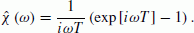((11))

By considering this source time function (11), we can use the residue theorem for integrating with respect to ω in Eq. (6). Substituting Eq. (11) into Eq. (6) and recognizing that poles are located at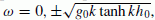, we calculated Eq. (6) as:((12))

where: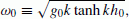((13))

(Appendix A). Equation (13) represents the dispersion relation for water waves (e.g., Stoker, 1958) and the corresponding phase velocity is given by:((14))

where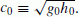.

Introducing the following function:((15))

we can rewrite Eq. (12) as follows:((16))

Equations (15) and (16) give the solution of the velocity potential for tsunami generation propagation when the sea-bottom deformation is represented by Eqs. (4) and (9).

### 2.3 A general solution: impulse response

Assuming that the duration T in Eq. (16) approaches zero, we obtain the solution for instantaneous sea-bottom deformation, or for the impulse response of the source time function given by χ (t) = δ (t). The solution is obtained as follows:((17))

By convoluting an arbitrary function χ (t) with Eq. (17), we now obtain the solution of the velocity potential with respect to the function generally given by Eq. (4) as follows:((18))

We can confirm that we obtain an equation equivalent to Eq. (12) if we substitute Eq. (9) into Eq. (18). Furthermore, if we assume thatin Eq. (17), we obtain the solution of the impulse response for the point source at the bottom,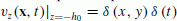as: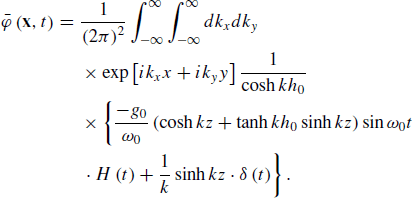((19))

Using Eq. (17), we obtain the horizontal components of the velocity field v H = v x e x + v y e y , the vertical component of the velocity v z , and the height of the sea surface η for an instantaneous sea-bottom deformation, or the velocity at the bottom is given by the delta function,as follows: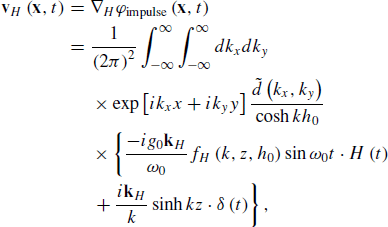((20))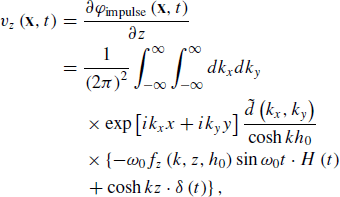((21))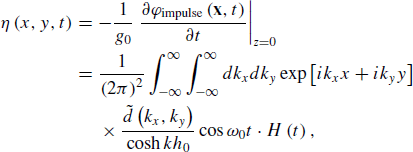((22))

where H is the gradient in the horizontal plane given by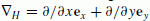, and k H is the wavenumber vector in the horizontal plane given by k H = k x e x + k y e y . Here, we introduce the distribution functions of the horizontal and vertical components of the velocity, as follows:((23))((24))

We discuss the meaning of these distribution functions (Eqs. (23) and (24)) together with the interpretations of Eqs. (20) through (22) in a later section (3.3 Propagation process). We can confirm that Eqs. (20) through (22) satisfy v = 0 and the boundary conditions (Eqs. (2) through (4)).

Ward (unpublished document available at http://es.ucsc. edu/̃ward/papers/Basic_Tsunami_Theory.doc) obtained an equation for displacement (equation (5.3.10) in the document) for an instantaneous sea-bottom deformation, whereas we have derived an expression for velocity (Eqs. (20) and (21)) in this study with the source term given by the delta function. The representation using the delta function is fundamentally important for investigating physical phenomena during tsunami generation. This enables us to discuss the initial velocity distribution in a 2-D numerical simulation (see Section 3.2: On the use of the depth filter or Kajiura’s equation) and the pressure change during tsunami generation (see Section 2.4: Pressure at the sea bottom during the tsunami generation), in particular, for an arbitrary source time function characterized by a finite source duration.

Ocean-bottom pressure gauges are often used for recording tsunamis (e.g., Tang et al., 2009). The pressure in the ocean is given by the sum of the hydrostatic pressure and an excess pressure due to the tsunami, i.e., −ρ0g0z + p e , where the excess pressure is given by the velocity potential as. Using the velocity potential of Eq. (17), we obtain the excess pressure brought about at the sea bottom by the tsunami as follows:((25))

for an impulse response. Equation (25) is similar, but not identical, to that obtained by Dutykh and Dias (2007) and Kervella et al. (2007), who derived the pressure at the sea bottom after an instantaneous sea-bottom uplift. However, they did not consider a source term, so the pressure change during the source process time cannot be inclusive. On the other hand, Eq. (25) includes an additional term (the second term in brackets {} in Eq. (25)) as a source term, which represents the contribution from a dynamic source process. We refer to this term as the dynamic source term hereinafter in this manuscript. Equation (25) indicates that the excess pressure due to the tsunami is not simply given by a static contribution from the tsunami height. Only when the wavelength is long enough compared with the water depth kh0 1 and the dynamic source term is neglected, can the excess pressure due to the tsunami be approximated by p e ρ0g0η. We investigate the contribution from the dynamic source term in the following.

### 2.4 Pressure at the sea bottom during tsunami generation

Ocean-bottom pressure gauges deployed near the hypocenter, or inside the source region, would play an important role for a rapid source estimation in an early tsunami warning (Tsushima et al., 2009, 2011, 2012). It is crucially important to provide a representation of the pressure at the sea bottom, in particular, for an arbitrary source of duration χ (t). For the source given by (4), we obtain: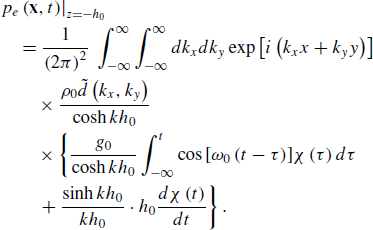((26))

In the derivation of Eq. (26), integrating by part, we calculate the time derivative of the delta function in Eq. (25) as the time derivative of the source time function in Eq. (26). This dynamic source term leads to an increase in excess pressure at the sea bottom when the sea bottom uplifts at an increasing rate d χ /dt > 0.

We roughly examine how the dynamic source term contributes to the total ocean-bottom pressure during tsunami generation. Considering a simple case where the source size is large enough, kh0 1, and focusing on tsunami generation, neglecting propagation, and simplifying the expression as cos [ω0 (tτ)] → 1, we approximate Eq. (26) as:((27))

In addition, assuming that the sea bottom uplifts with a constantly increasing rate d χ /dt = α0 (> 0) during the source process time from 0 to T, and χ (t) = 0 when t > T, we further simplify the first term in the brackets in Eq. (27) as, which should be equal to 1 at t = T (i.e. α0 = 2/T2, andwhen t < T). The second term (the dynamic source term) is then given by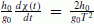. The first termincreases with elapsed time, whereas the dynamic source termis constant during the source process time 0 ≤ tT. The contribution from the dynamic source term to the total sea-bottom pressure at t = T is then given by. The contribution from the dynamic source term increases as the sea depth h0 increases, and as the source process time T decreases. Figure 3 plots the contribution from the dynamic source term as a function of the source process time T, which indicates that the contribution from the dynamic source term can vary widely from 2% to 90% depending on the time T and the sea depth. For example, supposing that the maximum tsunami height occurred where the sea depth was ~3000 m and the corresponding rise time was ~40 s as was the case in the 2011 Tohoku-Oki earthquake (Suzuki et al., 2011), we estimated that the dynamic source term contributes ~30%. Equations (25) and (26) should be used for more realistic situations, such as highly heterogeneous slip distributions and arbitrary source time functions (e.g. Geist, 2002). We can, at least, conclude that we cannot always neglect the dynamic source term. This is quite important for developing a rapid tsunami source estimation technique using ocean-bottom pressure gauges inside the source region.

## 3. Interpreting Tsunami Generation and Propagation

### 3.1 Generation process

For convenience, we consider the tsunami generation and propagation processes separately. We hereinafter refer to the process in which a sea-bottom deformation generates an initial tsunami-height distribution as the generation process, and the process in which the tsunami propagates from the initial tsunami-height distribution as the propagation process (Fig. 4(a)). In contrast to this situation, if we set the initial tsunami-height distribution η0(x, y) as shown in Fig. 4(b), we simulate only the propagation process and not the generation process. By comparing these two situations and finding differences, we intend to examine the generation process. The wavefields from the initial tsunami-height distribution η0(x, y) are given by (e.g., Kervella et al. 2007):((28))((29))((30))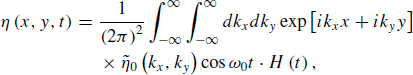((31))

where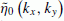is the 2-D spatial Fourier transform of η0(x, y).

Comparing Eqs. (20) through (22) and Eqs. (29) through (31), we recognize two differences. We may consider that the two points are peculiarities of the generation process. First,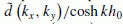appears in Eqs. (20) through (22) instead of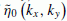as in Eqs. (29) through (31). The factor 1/cosh kh0 has an effect that is sometimes referred to as a depth filter (e.g., Saito and Furumura, 2009; Tsushima et al., 2012), and the corresponding equation in the spatial domain is often referred to as Kajiura’s equation (e.g., Kajiura, 1963; Tanioka and Seno, 2001). This is a filtering effect from the sea bottom to the sea surface for an instantaneous sea-bottom deformation (e.g. Ward, 2001). The sea-bottom deformation in the wavenumber domain given by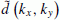is transmitted to the sea surfaceat a constant water depth of h0 in the generation process (e.g. Takahashi, 1942; Ward, 2001; Dutykh et al., 2006; Dutykh and Dias, 2007; Kervella et al., 2007).

Secondly, Eqs. (20) and (21) each have a term that includes the delta function, whereas Eqs. (29) and (30) do not. These terms come from the term that includes the delta function in Eq. (17), which represents a source term originating from the sea-bottom deformation. Figure 5 shows the amplitudes of the horizontal components of the source terms (proportional to ǀ sinh kz ǀ, see Eq. (20)) and their vertical components (proportional to ǀcosh kzǀ, see Eq. (21)) normalized by the vertical component at the sea bottom (proportional to ǀcosh kh0ǀ, see Eq. (21)) for the values of k = 0.1 and 0.3 km−1 corresponding to wavelengths of 60 and 20 km for a water depth of 4 km. The vertical component decreases more gradually as the wavelength increases from 20 to 60 km. The horizontal component relative to the vertical component at the bottom of the 20-km wavelength is larger than that when the wavelength is 60 km. This indicates that a larger source more efficiently excites the vertical flow, while a smaller source less inefficiently due to horizontal flow excitation.

### 3.2 On the use of a depth filter or Kajiura’s equation

The initial tsunami-height distribution is not always equivalent to the sea-bottom deformation, particularly, for a steep sea-bottom deformation. In order to evaluate the initial tsunami-height distribution from the sea-bottom deformation, Kajiura’s equation (e.g., Tanioka and Seno, 2001) or a depth filter (e.g., Tsushima et al., 2012) is used to make the correction. When using this corrected initial tsunami-height distribution in 2-D numerical tsunami simulations, the initial velocity distribution is usually assumed to be zero. Ward (unpublished note available at http://es.ucsc.edu/̃ward/papers/Basic_Tsunami_Theory. doc) proved that a tsunami propagating from a bottom displacement is identical to one from the same initial tsunami-height distribution, except for the depth filter correction when the sea bottom uplifts instantaneously. We consider here an arbitrary source time function of χ (t) and examine the initial conditions for 2-D numerical tsunami propagation simulations using Eqs. (20) and (21).

Both Eqs. (20) and (21) appear to indicate that a nonzero velocity distribution exists due to both the first and the second terms in the brackets {}. When considering the source time function χ (t), Eq. (20) becomes((32))

Since the second term in Eq. (20) is represented by the delta function, the second term becomes the source time function χ (t) in Eq. (32). On one hand, the first term contains a function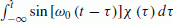. When we assume that the source time function χ (t) is non-zero only when t < T and is zero when t > T, the second term in the brackets {} in Eq. (32) becomes zero and only the first term contributes to the velocity distribution when t > T. If we compare Eq. (32) with Eq. (29), we may suppose that the first term in the bracket {} in Eq. (32) is generated from the water height distribution. In other words, we may say that the velocity distribution for t > T is generated only through the water height distribution. Note that the same thing holds for the vertical flow (see Eq. (21)).

Therefore, in order to properly include the tsunami generation process in the initial tsunami height distribution for 2-D tsunami simulations, only the effect of the water height distribution (the first term in Eq. (32)) is taken into account at each time step of the tsunami simulation. We should not add the velocity distribution generated from the source. This procedure is the same as that usually used in past studies for 2-D tsunami simulations (e.g., Fujii and Satake, 2008; Saito and Furumura, 2009). Equation (32) is a fundamental equation for validating the method for an arbitrary source time function.

### 3.3 Propagation process

Although the propagation process has been thoroughly investigated in numerous studies (e.g. Lamb, 1932; Leblond and Mysak, 1978; Mei, 1989; Ward, 2001), we summarize it in this section from the viewpoint of our equations. The distribution functions of the horizontal and vertical components of the velocity (Eqs. (23) and (24)) are common in Eqs. (20) and (21) and Eqs. (29) and (30). Hence, the terms that include the distribution functions are peculiar to the propagation process. The distribution functions of Eqs. (23) and (24) indicate the velocity distributions for the horizontal and vertical components, respectively, over a depth z in water of a constant depth h0, where the value is normalized at the surface, z = 0. Figure 6(a) graphs the distribution function of the horizontal component velocity (Eq. (23)) for values of k = 6.3, 1.6, and 0.1 km−1 corresponding to wavelengths of 1, 4, and 60 km for a water depth of 4 km. When the wavelength is small (λ = 1 km), the horizontal component of the velocity distributes only in the shallower regions (z < 1 km). As the wavelength increases, the distribution function extends deeper. When the wavelength is much larger than the water depth (λ = 60 km h0 = 4 km), the horizontal velocity component is distributed almost uniformly over the entire depth. Figure 6(b) indicates the function for the vertical component velocity (Eq. (24)). As in the case of the horizontal components, when the wavelength is small (λ = 1 km), the vertical component of the velocity is distributed only in the shallower region (z < 1 km). Unlike the case of the horizontal components, when the wavelength is much larger than the water depth (λ = 60 km), the vertical component of the velocity does not distribute uniformly, but decreases with increasing depth, and the value becomes zero at the sea bottom. This indicates that the vertical velocity component is zero at the sea bottom for tsunami propagation.

## 4. Examples

Numerous studies have investigated water height at the sea surface for tsunami generation and propagation processes (e.g., Kajiura, 1963; Hammack, 1973; Kervella et al., 2007; Dutykh et al., 2013). However, few studies have visualized the vertical cross-section of the velocity distribution, which is critically important to both tsunami generation and dispersive propagation. We visualize the velocity distribution and the height of the sea surface using fast Fourier transforms (FFT) for tsunami generation and propagation.

As a simple example, we assume tsunami generation for a constant-rate sea-bottom deformation, the boundary condition of which is given by:((33))

Substituting the corresponding Fourier transform ofinto Eqs. (20) through (22) and convoluting the corresponding source time function, we obtain the solution and plot the spatial and temporal water height and velocity distribution for the following two cases.

### Large source

We consider the case in which the vertical component of the velocity at the sea bottom is given by Eq. (32) with d0 = 1 m, a = 30 km, and T = 31 s. This source is sufficiently large compared with the sea depth, L = 2a (=60 km) > 13h0 (=52 km), when we use the criteria given by Saito and Furumura (2009). Figure 7 show the water height at the surface (upper panel) and the velocity distribution from the sea bottom to the sea surface (lower panel). During the source process time (T < 31 s), the vertical velocity at the sea bottom produces a vertical flow through the water over the source region (Figs. 7(a), 7(b), and 7(c)). If we look carefully, there is a horizontal velocity component (Fig. 7(a)). The horizontal velocity is larger nearer the bottom and approaches zero as shown in Fig. 5. As the source process time ends (T = 31 s), the vertical velocity provided by the sea bottom disappears. The collapse of the uplifted sea surface accelerates and causes a descending flow (Fig. 7(d)). Descending water displaces the water sideways from the source region and the water moves with a dominant horizontal flow (Figs. 7(e) and 7(f)). The horizontal velocity is always positive (negative) in horizontal locations where x is positive (negative). We see a small vertical component of the velocity near the surface, whereas no vertical component of the velocity exists near the bottom. The distribution functions (Fig. 6) also show these features when the wavelength is long (λ = 60 km).

### Small source

We then consider the case when a small source is given by L = 2a (=20 km) and T = 31 s (Fig. 8). During the source process time (T < 31 s), the vertical velocity from the sea bottom produces a vertical flow of water over the source region (Figs. 8(a), 8(b), and 8(c)). However, the maximum water height at the surface (0.8 m) is smaller than in the case of the larger source. This is interpreted that both the water depth and the source duration work as a kind of low-pass filter in space for the tsunami generation process (e.g., Saito and Furumura, 2009). Also, we may interpret this as showing that the vertical velocity is not efficiently excited by the excitation of the horizontal flow, for a small source such as is shown in Fig. 5. After the source process time ends (T > 31 s), the collapse of the uplifted sea surface accelerates, which causes a descending flow (Fig. 8(d)). The descending water displaces the water sideways, and the water moves with a horizontal flow. Unlike the case of a large source, the vertical component of the velocity is also significant (Figs. 8(e) and 8(f)). The water height at the surface indicates a dispersive wave. A short-wavelength tsunami propagates more slowly than a long-wavelength one. The horizontal component of the velocity distribution corresponding to the dispersive wave oscillates between positive and negative values. Moreover, prograde-rotation of the velocity vector occurs near the surface. The velocity distribution accompanied by the short-wavelength tsunami is localized near the surface (Fig. 8(f)). This is consistent with the distribution functions for a short-wavelength case (Fig. 6). These phenomena relating to the dispersive wave are unique to deep waves or short-wavelength tsunamis.

## 5. Concluding Remarks

We have presented a new solution for the velocity potential in the time domain (Eqs. (17) and (18)) for an arbitrary sea-bottom deformation. Using this velocity potential, we can represent the spatial and temporal variation of the pressure at the sea bottom (Eq. (25)), the velocity distributions in the sea (Eqs. (20) and (21)), and the water height at the sea surface (Eq. (22)).

The solution for the ocean-bottom pressure (Eqs. (25) and (26)) indicates that when the sea bottom uplifts at an increasing rate, the sea-bottom pressure increases dynamically. This dynamic contribution will not be negligible as we develop more rapid and precise source estimation techniques using ocean-bottom pressure gauges within the source region (Figs. 1 and 3). The velocity distribution is represented by the sum of the direct and indirect components excited by the sea-bottom deformation (Eq. (32)): the direct component is the velocity distribution excited directly by the sea-bottom deformation, while the indirect component is the velocity distribution excited by the waterheight distribution at the surface. The solution indicates that the direct component should be zero, or the initial velocity distribution should be zero at the initial conditions for 2-D tsunami propagation simulations. Using the solutions of Eqs. (20)2)sualize and interpret the spatial and temporal distributions of both the water height and the velocity vector in the sea from the sea-bottom deformation including, in particular, the finite duration of the source process time. We can interpret the generation and propagation using the amplitude distributions shown in Figs. 5 and 6.

The equations obtained in this study (Eqs. (17), (20)through (22), and (25)) are fundamental equations that constitute a mathematical basis for describing tsunami generation and propagation in incompressible water. These equations enable us to interpret and analyze the records of the tsunami velocity field through the water and the records of the ocean-bottom pressure, whereas conventional equations cannot. In particular, from the ocean-bottom pressure records within the source region, it is possible to extract information relating to the acceleration of the sea-bottom deformation, which is directly related to the dynamics of the earthquake fault rupturing. However, since actual records also contain acoustic waves (e.g. Yamamoto, 1982; Nosov and Kolesov, 2007; Maeda et al., 2011, 2013) that cannot be modeled by the incompressible theory assumed in this study, it is necessary to filter these out before applying the equations of the present study to the observed records. It is important to develop the equations for the case of compressible flow for future studies. Nevertheless, the analytical solutions obtained herein can be used to validate simulation codes because, at present, a number of numerical simulations assume an incompressible flow. Moreover, the solutions for an incompressible flow will serve as a sound basis for investigating the contribution of compressible flow in future studies.

## References

• Cui, H., J. D. Pietrzak, and G. S. Stelling, A finite volume analogue of the P1NC-P1 finite element: With accurate flooding and drying Ocean Modeling, 35, 16–30, doi:10.1016/j.ocemod.2010.06.001, 2010.

• Dutykh D. and F. Dias Water waves generated by a moving bottom in Tsunami and Nonlinear Waves edited by A. Kundu Springer Verlag 2007.

• Dutykh D. F. Dias and Y. Kervella Linear theory of wave generation by a moving bottom, C. R. Acad. Sci. Paris, Ser. I, 343, 499–504, 2006.

• Dutykh D. D. Mitsotakis X. Gardeil and F. Dias On the use of the finite fault solution for tsunami generation problems Theor. Comput. Fluid Dyn. 27 177–199 2013.

• Fujii, Y. and K. Satake Tsunami sources of the November 2006 and January 2007 great Kuril earthquakes Bull. Seismol. Soc. Am. 98 1559–1571 doi:10.1785/0120070221 2008.

• Geist E. L. Complex earthquake rupture and local tsunamis J. Geophys. Res., 107 doi:10.1029/2000JB000139 2002.

• Grilli S. T. S. Vogelmann and P. Watts Development of a 3D numerical wave tank for modeling tsunami generation by underwater landslides Eng. Anal. Bound. Elem., 26 301–313 2002.

• Hammack J. L. A note on tsunamis: Their generation and propagation in an ocean of uniform depth. J. Fluid Mech., 60 769–799 1973.

• Ito, Y., T. Tsuji, Y. Osada, M. Kido, D. Inazu, Y. Hayashi, H. Tsushima, R. Hino, and H. Fujimoto, Frontal wedge deformation near the source region of the 2011 Tohoku-Oki earthquake, Geophys. Res. Lett., doi:10.1029/2011GL048355, 2011.

• Kajiura, K., The leading wave of a tsunami, Bull. Earthq. Res. Inst., 41, 545–571, 1963.

• Kakinuma, T. and M. Akiyama, Numerical analysis of tsunami generation due to seabed deformation, Doboku Gakkai Ronbunshuu B, 62, 388–405, 2006 (in Japanese with English abstract).

• Kanazawa, T., Japan Trench earthquake and tsunami monitoring network of cable-linked 153 ocean bottom observatories and its impact to earth disaster science (UT2013-1147), International Symposium on Underwater Technology 2013, 2013.

• Kanazawa, T. and M. Shinohara, A new, compact ocean bottom cabled seismometer system—Development of compact cabled seismometers for seafloor observation and a description of first installation plan, Sea Technology, 50(7), 37–40, 2009.

• Kervella, Y., D. Dutykh, and F. Dias, Comparison between three-dimensional linear and nonlinear tsunami generation models, Theor. Comput. Fluid Dyn., 21, 245–269, 2007.

• Lamb, H., Hydrodynamics, 6th ed., Dover Publications, 1932.

• Leblond, P. H. and L. A. Mysak, Waves in the Ocean, Elsevier, 1978.

• Levin, B. W. and M. A. Nosov, Physics of Tsunamis, Springer, 2009.

• Liu, P. L.-F., Tsunami modeling—propagation, in The Sea, edited by A. R. Robinson and E. N. Bernard, pp. 295–319, Harvard University Press, Cambridge, Massachusetts, 2009.

• Mader, C. L., Numerical Modeling of Water Waves, CRC Press, Boca Raton, N. Y, 2004.

• Maeda, T. and T. Furumura, FDM simulation of seismic waves, ocean acoustic waves, and tsunamis based on tsunami-coupled equations of motion, Pure Appl. Geophys., 170(1–2), 109–127, doi:10.1007/s00024-011-0430-z, 2013.

• Maeda, T., T. Furumura, S. Sakai, and M. Shinohara, Significant tsunami observed at ocean-bottom pressure gauges during the 2011 off the Pacific coast of Tohoku Earthquake, Earth Planets Space, 63, doi:10.5047/eps.2011.06.005, 2011.

• Maeda, T., T. Furumura, S. Noguchi, S. Takemura, S. Sakai, M. Shinohara, K. Iwai, and S.-J. Lee, Seismic- and tsunami-wave propagation of the 2011 Off the Pacific Coast of Tohoku Earthquake as inferred from the tsunami-coupled finite-difference simulation, Bull. Seismol. Soc. Am., 103(2B), 1456–1472, doi:10.1785/0120120118, 2013.

• Mei, C. C., The Applied Dynamics of Ocean Surface Waves, World Scientific Publishing Co. Pte. Ltd., 1989.

• Monastersky, R., The next wave, Nature, 483, 144–146, doi:10.1038/483144a, 2012.

• Nosov, M. A. and S. V. Kolesov, Elastic oscillations of water column in the 2003 Tokachi-oki tsunami source: In-situ measurements and 3-D numerical modeling, Nat. Haz. Earth Syst. Sci., 7, 243–249, doi:10.5194/nhess-7-243-2007, 2007.

• Obara, K., K. Kasahara, S. Hori, and Y. Okada, A densely distributed high-sensitivity seismograph network in Japan: Hi-net by National Research Institute for Earth Science and Disaster Prevention, Rev. Sci. Instrum., 76, 021301, doi:10.1063/l.1854197, 2005.

• Ohmachi, T., H. Tsukiyama, and H. Matsumoto, Simulation of tsunami induced by dynamic displacement of seabed due to seismic faulting, Bull. Seismol. Soc. Am., 91, 1898–1909, 2001.

• Okada, Y., K. Kasahara, S. Hori, K. Obara, S. Sekiguchi, H. Fujiwara, and A. Yamamoto, Recent progress of seismic observation networks in Japan—Hi-net, F-net, K-NET, and KiK-net, Earth Planets Space, 56, xv–xxviii, 2004.

• Saito, T. and T. Furumura, Three-dimensional tsunami generation simulation due to sea-bottom deformation and its interpretation based on the linear theory, Geophys. J. Int., 178, 877–888, doi:10.1111/j.1365-246X.2009.04206.x, 2009.

• Saito, T., K. Satake, and T. Furumura, Tsunami waveform inversion including dispersive waves: The 2004 earthquake off Kii Peninsula, Japan, J. Geophys. Res., 115, B06303, doi:10.1029/2009JB006884, 2010.

• Saito, T., Y. Ito, D. Inazu, and R. Hino, Tsunami source of the 2011 Tohoku-Oki earthquake, Japan: Inversion analysis based on dispersive tsunami simulations, Geophys. Res. Lett., 38, L00G19, doi:10.1029/2011GL049089, 2011.

• Sato, M., T. Ishikawa, N. Ujihara, S. Yoshida, M. Fujita, M. Mochizuki, and A. Asada, Displacement above the hypocenter of the 2011 Tohokuk-Oki Earthquake, Science, 332, 1395, doi:10.1126/science.1207401, 2011.

• Shuto, N., Numerical simulation of tsunamis—Its present and near future, Nat. Hazards, 4, 171–191, doi:10.1007/BF00162786, 1991.

• Stoker, J. J., Water Waves: The Mathematical Theory with Applications, John Wiley and Sons, Inc., 1958.

• Suzuki, W., S. Aoi, H. Sekiguchi, and T. Kunugi, Rupture process of the 2011 Tohoku-Oki mega-thrust earthquake (M9.0) inverted from strong-motion data, Geophys. Res. Lett., 38, L00G16, doi:10.1029/2011GL049136, 2011.

• Takahashi, R., On seismic sea waves caused by deformations of the sea bottom, Bull. Earthq. Res. Inst., 20, 357–400, 1942 (in Japanese with English abstract).

• Tang, L., V. V. Titov, and C. D. Chamberlin, Development, testing, and applications of site-specific tsunami inundation models for real-time forecasting, J. Geophys. Res., 114, C12025, doi:10.1029/2009JC005476, 2009.

• Tanioka, Y. and T. Seno, Sediment effect on tsunami generation of the 1896 Sanriku tsunami earthquake, Geophys. Res. Lett., 28, 3389–3392, 2001.

• Tsushima, H., R. Hino, H. Fujimoto, Y. Tanioka, and F. Imamura, Near-field tsunami forecasting from cabled ocean bottom pressure data, J. Geophys. Res., 114, B06309, doi:10.1029/2008JB005988, 2009.

• Tsushima, H., K. Hirata, Y. Hayashi, Y. Tanioka, K. Kimura, S. Sakai, M. Shinohara, T. Kanazawa, R. Hino, and K. Maeda, Near-field tsunami forecasting using offshore tsunami data from the 2011 off the Pacific coast of Tohoku Earthquake, Earth Planets Space, 63, 821–826, 2011.

• Tsushima, H., R. Hino, Y. Tanioka, F. Imamura, and H. Fujimoto, Tsunami waveform inversion incorporating permanent seafloor deformation and its application to tsunami forecasting, J. Geophys. Res., 117, B03311, doi:10.1029/2011JB008877, 2012.

• Uehira, K., T. Kanazawa, S. Noguchi, S. Aoi, T. Kunugi, T. Matsumoto, Y. Okada, S. Sekiguchi, K. Shiomi, and T. Yamada, Ocean bottom seismic and tsunami network along the Japan Trench, AGU Fall Meeting, OS41C-1736, 2012.

• Ward, S. N., Tsunamis, in The Encyclopedia of Physical Science and Technology, edited by R. A. Meyers, Academic, San Diego, Calif, 2001.

• Yamamoto, T., Gravity waves and acoustic waves generated by submarine earthquakes, Soil Dyn. Earthq. Eng., 1, 75–82, 1982.

## Acknowledgments

The author would like to thank D. Inazu, T. Maeda, S. Ward, T. Hara, and an anonymous reviewer for careful reading and constructive comments.

## Author information

Authors

### Corresponding author

Correspondence to Tatsuhiko Saito.

## Appendix A. Calculation of Eq. (12)

### Appendix A. Calculation of Eq. (12)

An artificial damping parameter ε1 is introduced in Eq. (2) as: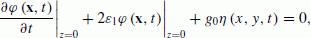((A.1))

and also another damping parameter ε2 in Eq. (9) as:((A.2))

These artificial damping parameters will be taken as zero. When the Eqs. (A.1) and (A.2) are used, the equation corresponding to Eq. (12) is written as: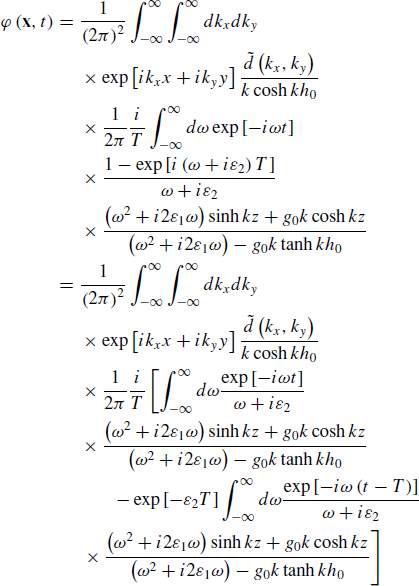((A.3))

Considering that the artificial damping is very small, or that the artificial damping parameters are much smaller than the angular frequency for tsunami, we employ the residue theorem. The poles of Eq. (A.3) are located at ω = −iε1 ± ω0 and −iε2 in the lower half of the ω-plane (Fig. A.1), where(see Eq. (13)). When tT < 0, we take the path of the integral in the upper half plane (path I in Fig. A.1) including no poles. When tT > 0, we take the integral path in the lower half plane including the poles (path II in Fig. A.1). The second term in the bracket in Eq. (A.3), for example, is then calculated as: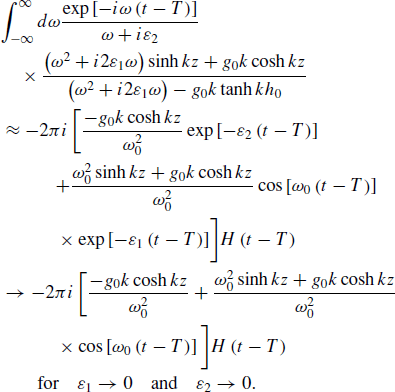((A.4))

In a similar way, the first term in the bracket in Eq. (A.3) can also be calculated. The velocity potential (A.3) is then given by: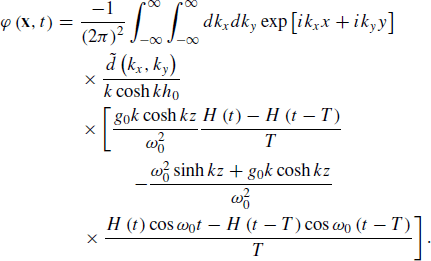((A.5))

If Eq. (13) is used, Eq. (A.5) is confirmed to be identical to Eq. (12).

## Rights and permissions

The images or other third party material in this article are included in the article’s Creative Commons licence, unless indicated otherwise in a credit line to the material. If material is not included in the article’s Creative Commons licence and your intended use is not permitted by statutory regulation or exceeds the permitted use, you will need to obtain permission directly from the copyright holder.

To view a copy of this licence, visit https://creativecommons.org/licenses/by/4.0/.

Reprints and Permissions

Saito, T. Dynamic tsunami generation due to sea-bottom deformation: Analytical representation based on linear potential theory. Earth Planet Sp 65, 1411–1423 (2013). https://doi.org/10.5047/eps.2013.07.004

• Revised:

• Accepted:

• Published:

• Issue Date:

• DOI: https://doi.org/10.5047/eps.2013.07.004

### Key words

• Tsunami
• theory
• generation
• propagation